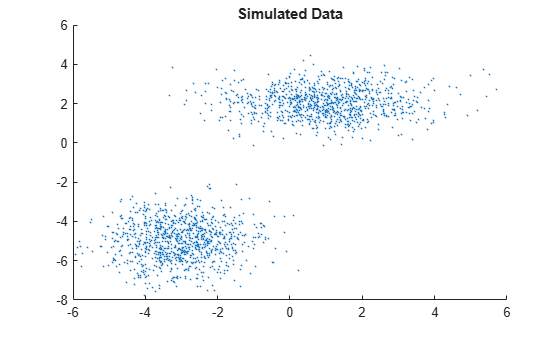Documentation

### This is machine translation

Mouseover text to see original. Click the button below to return to the English version of the page.

Note: This page has been translated by MathWorks. Click here to see
To view all translated materials including this page, select Country from the country navigator on the bottom of this page.

## Fit Gaussian Mixture Model to Data

This example shows how to simulate data from a multivariate normal distribution, and then fit a Gaussian mixture model (GMM) to the data using `fitgmdist`. To create a known, or fully specified, GMM object, see Create Gaussian Mixture Model.

`fitgmdist` requires a matrix of data and the number of components in the GMM. To create a useful GMM, you must choose `k` carefully. Too few components fails to model the data accurately (i.e., underfitting to the data). Too many components leads to an over-fit model with singular covariance matrices.

Simulate data from a mixture of two bivariate Gaussian distributions using `mvnrnd`.

```mu1 = [1 2]; sigma1 = [2 0; 0 .5]; mu2 = [-3 -5]; sigma2 = [1 0; 0 1]; rng(1); % For reproducibility X = [mvnrnd(mu1,sigma1,1000); mvnrnd(mu2,sigma2,1000)];```

Plot the simulated data.

```scatter(X(:,1),X(:,2),10,'.') % Scatter plot with points of size 10 title('Simulated Data')```Fit a two-component GMM. Use the `'Options'` name-value pair argument to display the final output of the fitting algorithm.

```options = statset('Display','final'); gm = fitgmdist(X,2,'Options',options)```
```5 iterations, log-likelihood = -7105.71 gm = Gaussian mixture distribution with 2 components in 2 dimensions Component 1: Mixing proportion: 0.500000 Mean: -3.0377 -4.9859 Component 2: Mixing proportion: 0.500000 Mean: 0.9812 2.0563 ```

Plot the pdf of the fitted GMM.

```gmPDF = @(x,y)reshape(pdf(gm,[x(:) y(:)]),size(x)); hold on h = fcontour(gmPDF,[-8 6]); title('Simulated Data and Contour lines of pdf');```Display the estimates for means, covariances, and mixture proportions

`ComponentMeans = gm.mu`
```ComponentMeans = 2×2 -3.0377 -4.9859 0.9812 2.0563 ```
`ComponentCovariances = gm.Sigma`
```ComponentCovariances = ComponentCovariances(:,:,1) = 1.0132 0.0482 0.0482 0.9796 ComponentCovariances(:,:,2) = 1.9919 0.0127 0.0127 0.5533 ```
`MixtureProportions = gm.ComponentProportion `
```MixtureProportions = 1×2 0.5000 0.5000 ```

Fit four models to the data, each with an increasing number of components, and compare the Akaike Information Criterion (AIC) values.

```AIC = zeros(1,4); gm = cell(1,4); for k = 1:4 gm{k} = fitgmdist(X,k); AIC(k)= gm{k}.AIC; end```

Display the number of components that minimizes the AIC value.

```[minAIC,numComponents] = min(AIC); numComponents```
```numComponents = 2 ```

The two-component model has the smallest AIC value.

Display the two-component GMM.

`gm2 = gm{numComponents}`
```gm2 = Gaussian mixture distribution with 2 components in 2 dimensions Component 1: Mixing proportion: 0.500000 Mean: -3.0377 -4.9859 Component 2: Mixing proportion: 0.500000 Mean: 0.9812 2.0563 ```

Both the AIC and Bayesian information criteria (BIC) are likelihood-based measures of model fit that include a penalty for complexity (specifically, the number of parameters). You can use them to determine an appropriate number of components for a model when the number of components is unspecified.

Download ebook